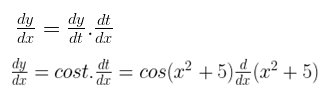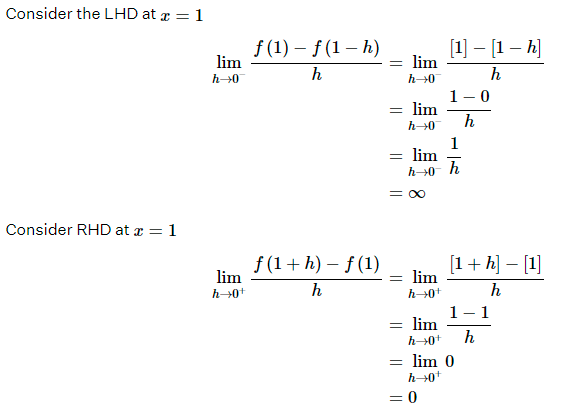NCERT Solutions for Maths Class 12 Exercise 5.2

# NCERT Solutions for Maths Class 12 Exercise 5.2

## NCERT Solutions for Maths Class 12 Exercise 5.2

Differentiate the functions with respect to x in Questions 1 to 8.

### Maths Class 12 Ex 5.2 Question 1.

sin (x² + 5)

Solution:
Let y = sin (x2 + 5),
Put x² + 5 = t
Then, y = sin t= cos (x² + 5) × 2x
= 2x cos (x² + 5)

### Maths Class 12 Ex 5.2 Question 2.

cos (sin x)

Solution:
Let y = cos (sin x)
Put sin x = t
y = cos t, where t = sin x

### Maths Class 12 Ex 5.2 Question 3.

sin (ax + b)

Solution:
Let y = sin (ax + b)
Put ax + b = t
y = sin t, where t = ax + b

### Maths Class 12 Ex 5.2 Question 4.

sec (tan (√x))

Solution:
Let y = sec (tan (√x))
By chain rule,

### Maths Class 12 Ex 5.2 Question 5.Solution:

### Maths Class 12 Ex 5.2 Question 6.

cos x³ . sin² (x5)

Solution:
Let y = cos x³ . sin² (x5)

### Maths Class 12 Ex 5.2 Question 7.cos (√x)

Solution:

### Maths Class 12 Ex 5.2 Question 9.

Prove that the function f given by f(x) = |x – 1|, x R is not differentiable at x = 1.

Solution:
We have f(x) = |x – 1|, x R

It is known that a function f is differentiable at a point x = c in its domain if both LHD and RHD are finite and equal.
To check the differentiability of the given function at x = 1,

Since LHD and RHD at x = 1 are not equal,

Therefore, f is not differentiable at x = 1.

### Maths Class 12 Ex 5.2 Question 10.

Prove that the greatest integer function defined by f(x) = [x], 0 < x < 3 is not differential at x = 1 and x = 2.

Solution:
We have f(x) = [x], 0 < x < 3

It is known that a function f is differentiable at a point x = c in its domain if both LHD and RHD are finite and equal.

To check the differentiability of the given function at x = 1,Since LHD and RHD at x = 1 are not equal,

Therefore, f is not differentiable at x = 1.

Similarly, f is not differentiable at x = 2.